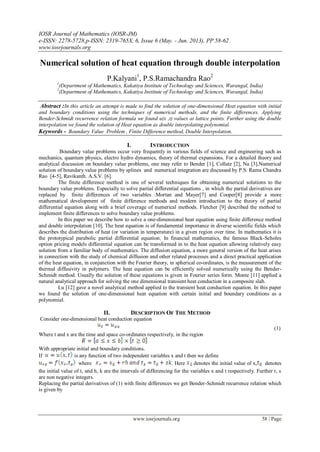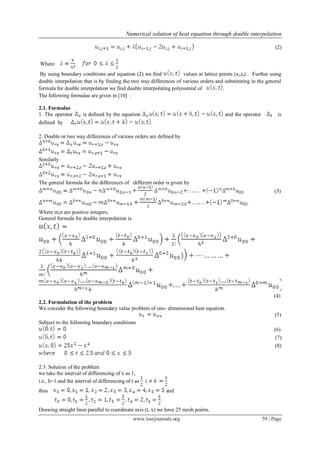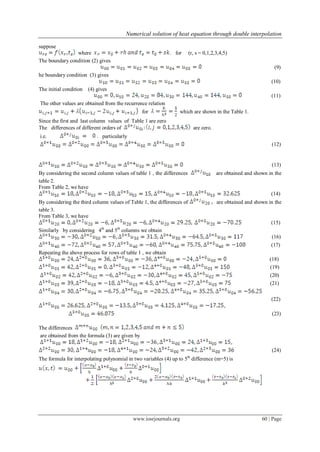Diese Präsentation wurde erfolgreich gemeldet.

# Numerical solution of heat equation through double interpolation

Anzeige
Anzeige
Anzeige
Anzeige
Anzeige
Anzeige
Anzeige
Anzeige
Anzeige
Anzeige
AnzeigeAnzeige
×

1 von 5 Anzeige

# Numerical solution of heat equation through double interpolation

In this article an attempt is made to find the solution of one-dimensional Heat equation with initial and boundary conditions using the techniques of numerical methods, and the finite differences. Applying Bender-Schmidt recurrence relation formula we found u(x ,t) values at lattice points. Further using the double interpolation we found the solution of Heat equation as double interpolating polynomial

In this article an attempt is made to find the solution of one-dimensional Heat equation with initial and boundary conditions using the techniques of numerical methods, and the finite differences. Applying Bender-Schmidt recurrence relation formula we found u(x ,t) values at lattice points. Further using the double interpolation we found the solution of Heat equation as double interpolating polynomial

Anzeige
Anzeige

### Numerical solution of heat equation through double interpolation

1. 1. IOSR Journal of Mathematics (IOSR-JM) e-ISSN: 2278-5728,p-ISSN: 2319-765X, 6, Issue 6 (May. - Jun. 2013), PP 58-62 www.iosrjournals.org www.iosrjournals.org 58 | Page Numerical solution of heat equation through double interpolation P.Kalyani1 , P.S.Ramachandra Rao2 1 (Department of Mathematics, Kakatiya Institute of Technology and Sciences, Warangal, India) 2 (Department of Mathematics, Kakatiya Institute of Technology and Sciences, Warangal, India) Abstract :In this article an attempt is made to find the solution of one-dimensional Heat equation with initial and boundary conditions using the techniques of numerical methods, and the finite differences. Applying Bender-Schmidt recurrence relation formula we found u(x ,t) values at lattice points. Further using the double interpolation we found the solution of Heat equation as double interpolating polynomial. Keywords - Boundary Value Problem , Finite Difference method, Double Interpolation. I. INTRODUCTION Boundary value problems occur very frequently in various fields of science and engineering such as mechanics, quantum physics, electro hydro dynamics, theory of thermal expansions. For a detailed theory and analytical discussion on boundary value problems, one may refer to Bender , Collatz , Na ,Numerical solution of boundary value problems by splines and numerical integration are discussed by P.S. Rama Chandra Rao [4-5], Ravikanth. A.S.V. . The finite difference method is one of several techniques for obtaining numerical solutions to the boundary value problems. Especially to solve partial differential equations , in which the partial derivatives are replaced by finite differences of two variables .Mortan and Mayer and Cooper provide a more mathematical development of finite difference methods and modern introduction to the theory of partial differential equation along with a brief coverage of numerical methods. Fletcher  described the method to implement finite differences to solve boundary value problems. In this paper we describe how to solve a one-dimensional heat equation using finite difference method and double interpolation . The heat equation is of fundamental importance in diverse scientific fields which describes the distribution of heat (or variation in temperature) in a given region over time. In mathematics it is the prototypical parabolic partial differential equation. In financial mathematics, the famous Black-Scholes option pricing models differential equation can be transformed in to the heat equation allowing relatively easy solution from a familiar body of mathematics. The diffusion equation, a more general version of the heat arises in connection with the study of chemical diffusion and other related processes and a direct practical application of the heat equation, in conjunction with the Fourier theory, in spherical co-ordinates, is the measurement of the thermal diffusivity in polymers. The heat equation can be efficiently solved numerically using the Bender- Schmidt method. Usually the solution of these equations is given in Fourier series form. Monte  applied a natural analytical approach for solving the one dimensional transient heat conduction in a composite slab. Lu  gave a novel analytical method applied to the transient heat conduction equation. In this paper we found the solution of one-dimensional heat equation with certain initial and boundary conditions as a polynomial. II. DESCRIPTION OF THE METHOD Consider one-dimensional heat conduction equation (1) Where t and x are the time and space co-ordinates respectively, in the region With appropriate initial and boundary conditions. If is any function of two independent variables x and t then we define where . Here denotes the initial value of x, denotes the initial value of t, and h, k are the intervals of differencing for the variables x and t respectively. Further r, s are non negative integers. Replacing the partial derivatives of (1) with finite differences we get Bender-Schmidt recurrence relation which is given by
2. 2. Numerical solution of heat equation through double interpolation www.iosrjournals.org 59 | Page (2) Where By using boundary conditions and equation (2) we find values at lattice points (xr,ts) . Further using double interpolation that is by finding the two way differences of various orders and substituting in the general formula for double interpolation we find double interpolating polynomial of . The following formulae are given in  . 2.1. Formulae 1. The operator is defined by the equation and the operator is defined by . 2. Double or two way differences of various orders are defined by Similarly The general formula for the differences of different order is given by (3) Where m,n are positive integers. General formula for double interpolation is (4) 2.2. Formulation of the problem We consider the following boundary value problem of one- dimensional heat equation. (5) Subject to the following boundary conditions (6) (7) (8) 2.3. Solution of the problem we take the interval of differencing of x as 1, i.e., h=1.and the interval of differencing of t as thus and Drawing straight lines parallel to coordinate axis (t, x) we have 25 mesh points.
3. 3. Numerical solution of heat equation through double interpolation www.iosrjournals.org 60 | Page suppose where . for (r, s = 0,1,2,3,4,5) The boundary condition (2) gives (9) he boundary condition (3) gives (10) The initial condition (4) gives (11) The other values are obtained from the recurrence relation for which are shown in the Table 1. Since the first and last column values of Table 1 are zero The differences of different orders of are zero. i.e. . particularly (12) (13) By considering the second column values of table 1 , the differences are obtained and shown in the table 2. From Table 2, we have (14) By considering the third column values of Table 1, the differences of are obtained and shown in the table 3. From Table 3, we have (15) Similarly by considering 4th and 5th columns we obtain (16) (17) Repeating the above process for rows of table 1 , we obtain (18) (19) (20) (21) (22) (23) The differences are obtained from the formula (3) are given by (24) The formula for interpolating polynomial in two variables (4) up to 5th difference (m=5) is
4. 4. Numerical solution of heat equation through double interpolation www.iosrjournals.org 61 | Page (25) Substituting the values of from the equations (12), (18) and (24) in equation (25) and simplifying we obtain . (26) The required interpolating polynomial of which is the approximate solution of heat equation (5) is given by equation (26). III. TABLES Table 1. Function Table. t x 0 1 2 3 4 5 0 0 24 84 144 144 0 ½ 0 42 84 114 72 0 1 0 42 78 78 57 0 3/2 0 39 60 67.5 39 0 2 0 30 53.5 49.5 33.75 0 5/2 0 26.625 39.75 43.5 24.75 0 Table 2. Difference Table 1. 24 → 18 42 → -18 → 0 → 15 42 → -3 → -18 → -3 → -3 → 32.625 39 → -6 → 14.625 → -9 → 11.625 30 → 5.625 → -3.375 26.625
5. 5. Numerical solution of heat equation through double interpolation www.iosrjournals.org 62 | Page Table 3. Difference Table2. 84 → 0 84 → -6 → -6 → -6 78 → -12 → 29.25 → -18 → 23.25 → -70.25 60 → 11.25 → -41.25 → -6.75 → -18 53.25 → -6.75 → -13.5 39.75 . IV. CONCLUSION Problems considered in thermodynamics and engineering may yield parabolic equations. usually the analytical solution of such equations are in Fourier series. In this we obtain the solution as a polynomial in two variables. REFERENCES  Bender.C.M and Orszag.S.A., Advanced mathematics methods for scientist and engineers ( McGraw-Hill. New York,1978).  Collatz, L.,The numerical treatment of differential equations ( Springer Verlag,Berlin,1996).  Na,T.Y., Computational methods in engineering boundary value problems (Academic Press, New York,1979).  Rama ChandraRao,P.S., Solution of a Class of Boundary Value Problems using Numerical Integration, Indian journal of mathematics and mathematical sciences.vol.2.No2,2006,pp.137-146.  Rama Chandra Rao,P.S., Solution of fourth order of boundary value problems using spline functions, Indian Journal of Mathematics and Mathematical Sciences.vol.2.No1,2006, pp.47-56  Ravikanth,A.S.V., Numerical treatment of singular boundary value problems, Ph.D. Thesis, National Institute of Technology, Warangal, India,2002.  K.W. Mortan and D.F. Mayers. Numerical solution of partial differential equations: An introduction ( Cambridge University press, Cambridge,England.1994).  Jeffery Cooper, Introduction to partial differential equations with matlab (Birkhauser, Bosten, 1998).  Clive A.J,Fletcher, Computational techniques for fluid dynamics (Springer-Verlag,Berlin,1998).  J.B. Scorborough, Numerical mathematical analysis ( Oxford and IBH Publishing Co. Pvt. Ltd.,1966)  F.de Monte, Transient heat conduction in one-dimensional composite slab: A natural analytic approach, International Journal of heat and mass transfer,Vol.43,2000,pp.3607-3619.  X Lu,P Tervola and M Viljanen, An efficient analytical solution to the transient heat conduction in one dimensional hollow composite cylinder, J.Phy.A.Math.Gen.Vol.38,2005,pp.10145-10155.